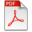# Identification of an LFT uncertainty model by minimizing the nu-gap metric

A4 Konferenspublikationer

## Interna författare/redaktörer

 Häggblom, Kurt Erik
Publikationens författare: Kurt E. Häggblom
Redaktörer: Anon
Förlagsort: New York, NY, USA
Publiceringsår: 2014
Förläggare: The Institute of Electrical and Electronics Engineers
Moderpublikationens namn: Proceedings of the 2014 European Control Conference (ECC)
Artikelns första sida, sidnummer: 246
Artikelns sista sida, sidnummer: 251
ISBN: 978-3-9524269-1-3

## Abstrakt

An uncertainty model in the form of a linear fractional transformation (LFT) is composed of a nominal model augmented by an uncertainty description. The size of the uncertainty required to cover a given set of models depends not only on the set of models, but also on the nominal model. Thus, the size of the uncertainty can be minimized by choosing the nominal model optimally according to some metric. If the uncertainty model is to be used for control design, a suitable metric is the nu-gap metric. It is shown that the optimal solution in terms of the nu-gap metric has to satisfy a bilinear matrix inequality (BMI) for every model in the model set. To solve this non-convex optimization problem, the BMIs are linearized to enable an iterative solution constrained by linear matrix inequalities (LMIs), where each iteration is a convex optimization problem. It is proved that the iteration converges to the optimal solution satisfying the BMIs. Because the solution is obtained as the frequency response at selected frequencies, the final model is determined by fitting a model to the frequency responses. A state-space model is used because the fitting can then easily be done subject to the same BMIs/LMIs to guarantee an optimal model. The procedure is illustrated by an application to uncertainty modeling of the product composition dynamics of a distillation column.

## Nyckelord

LMI/BMI/SOS techniques, Mathematical modeling, Nu-gap metric, System identification, Uncertain systems

## DokumentHaggblomFinal.pdf